# timeSpectrum

Time-averaged wavelet spectrum

## Syntax

``tavgp = timeSpectrum(fb,x)``
``tavgp = timeSpectrum(fb,cfs)``
``[tavgp,f] = timeSpectrum(___)``
``[___] = timeSpectrum(___,Name,Value)``
``timeSpectrum(___)``

## Description

````tavgp = timeSpectrum(fb,x)` returns the time-averaged wavelet power spectrum of the signal `x` using the continuous wavelet transform (CWT) filter bank `fb`. By default, `tavgp` is obtained by time-averaging the magnitude-squared scalogram over all times. The power of the time-averaged wavelet spectrum is normalized to equal the variance of `x`.```
````tavgp = timeSpectrum(fb,cfs)` returns the time-averaged wavelet spectrum for the CWT coefficients `cfs`. NoteWhen using this syntax, the power of the time-averaged wavelet spectrum is normalized to equal the variance of the last signal processed by the filter bank object function `wt`. ```

example

````[tavgp,f] = timeSpectrum(___)` returns the wavelet center frequencies or center periods for the time-averaged wavelet spectrum. `f` is a column vector or duration array depending on whether the sampling frequency or sampling period is specified in the CWT filter bank, `fb`.```

example

````[___] = timeSpectrum(___,Name,Value)` specifies additional options using name-value pair arguments. These arguments can be added to any of the previous input syntaxes. For example, `'Normalization','none'` specifies no normalization of the time-averaged wavelet spectrum.```
````timeSpectrum(___)` with no output arguments plots the time-averaged wavelet power spectrum in the current figure.```

## Examples

collapse all

Load the NPG2006 dataset . The data is the trajectory of a subsurface float trapped in an eddy. Plot the eastward and northward displacement. The triangle marks the initial position.

```load npg2006 plot(npg2006.cx) hold on grid on xlabel('Eastward Displacement (km)') ylabel('Northward Displacement (km)') plot(npg2006.cx(1),'^','markersize',11,'color','r',... 'markerfacecolor',[1 0 0 ])```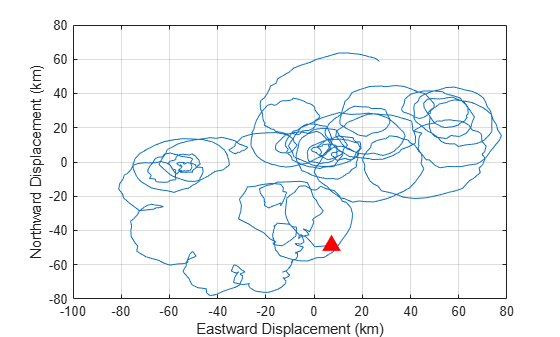Create a CWT filter bank that can be applied to the data. Use the default Morse wavelet. The sampling period for the data is 4 hours.

`fb = cwtfilterbank('SignalLength',length(npg2006.cx),'SamplingPeriod',hours(4));`

Obtain the time-averaged wavelet power spectra and the center periods.

```[tavgp,centerP] = timeSpectrum(fb,npg2006.cx); size(tavgp)```
```ans = 1×3 73 1 2 ```

The first page is the time-averaged wavelet spectrum for the positive scales (analytic part or counterclockwise component), and the second page is the time-averaged wavelet spectrum for the negative scales (anti-analytic part or clockwise component). Plot both spectra.

```subplot(2,1,1) plot(centerP,tavgp(:,1,1)) title('Counterclockwise Component') ylabel('Power') xlabel('Period (hrs)') subplot(2,1,2) plot(centerP,tavgp(:,1,2)) title('Clockwise Component') ylabel('Power') xlabel('Period (hrs)')```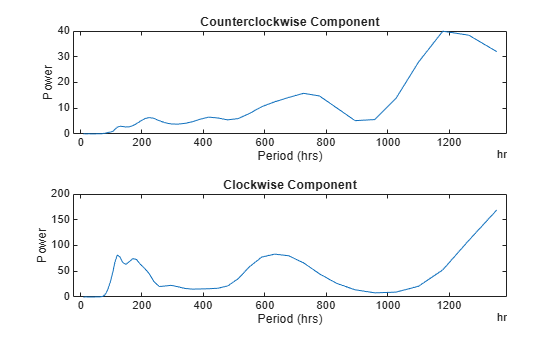If you omit the output arguments and execute `timeSpectrum(fb,npg2006.cx)` on the command line, the scalograms and time-averaged power spectra are plotted in the current figure. Note that the clockwise rotation of the float is captured in the clockwise rotary scalogram and the time-averaged spectrum.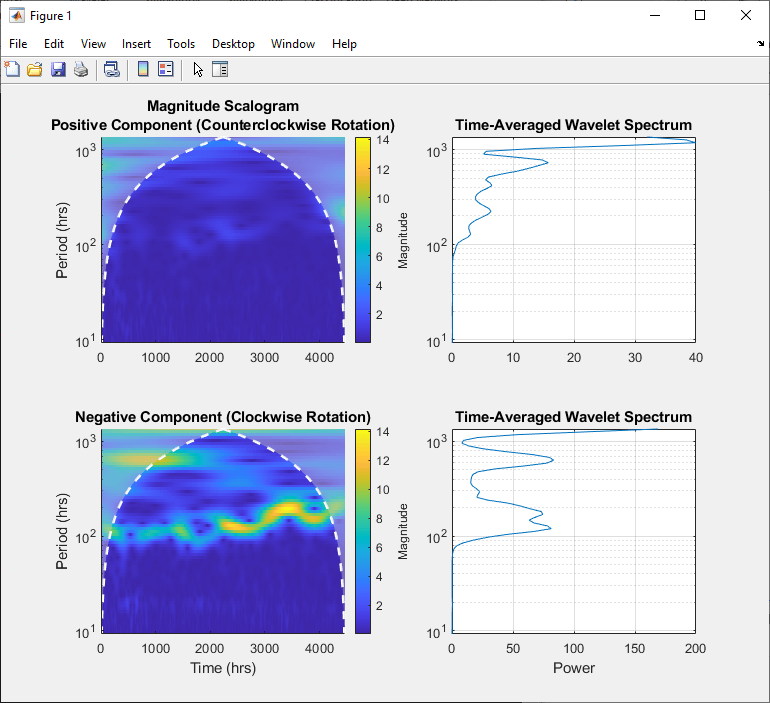Load a time series of solar magnetic field magnitudes recorded hourly over the south pole of the sun by the Ulysses spacecraft from 21:00 UT on December 4, 1993 to 12:00 UT on May 24, 1994. See  pp. 218–220 for a complete description of this data. Create a CWT filter bank that can be applied to the data. Plot the scalogram and the time-averaged wavelet spectrum.

```load solarMFmagnitudes fb = cwtfilterbank('SignalLength',length(sm),'SamplingPeriod',hours(1)); timeSpectrum(fb,sm)```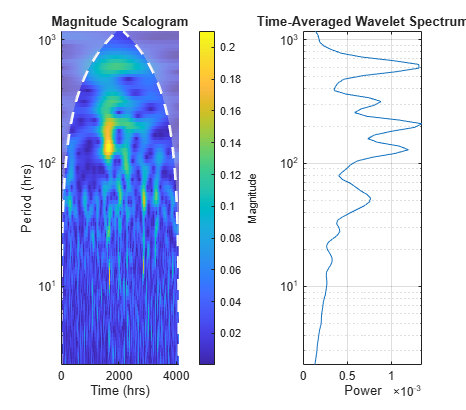Obtain the time-averaged wavelet spectrum of the signal using default values. By default, `timeSpectrum` normalizes the power of the time-averaged wavelet spectrum to equal the variance of the signal. Verify that the sum of the spectrum equals the variance of the signal.

```tavg = timeSpectrum(fb,sm); [var(sm) sum(tavg)]```
```ans = 1×2 0.0448 0.0447 ```

Obtain the time-averaged wavelet spectrum of the signal, but instead normalize the power as a probability density function. Verify that the sum is equal to 1.

```tavg = timeSpectrum(fb,sm,'Normalization','pdf'); sum(tavg)```
```ans = 1.0000 ```

If you set `SpectrumType` to `'density'`, `timeSpectrum` normalizes the weighted integral of the wavelet spectrum according to the value of `Normalization`. The spectrum mimics a probability density function whose integral, numerically evaluated, equals the value specified by `Normalization`.

Plot the scalogram and the time-averaged wavelet spectrum with spectrum type `'density'` and `'pdf'` normalization.

```figure timeSpectrum(fb,sm,'SpectrumType','density','Normalization','pdf')```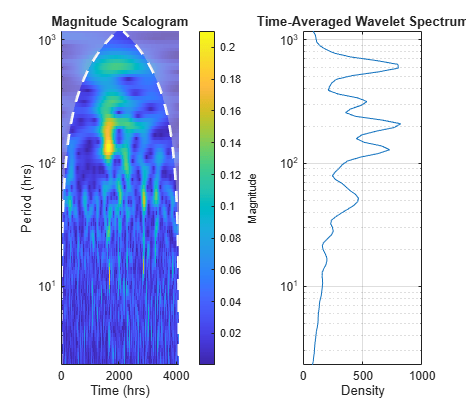To confirm the integral of the spectrum equals 1, first obtain the time-averaged wavelet spectrum with `'density'` spectrum type and `'pdf'` normalization.

`tavg = timeSpectrum(fb,sm,'SpectrumType','density','Normalization','pdf');`

By default, the filter bank uses the analytic Morse (3,60) wavelet. Obtain the admissibility constant for the wavelet and numerically integrate the wavelet spectrum using the trapezoidal rule. Keep in mind that the CWT uses L1 normalization. Confirm that the integral equals 1.

```ga = 3; tbw = 60; be = tbw/ga; anorm = 2*exp(be/ga*(1+(log(ga)-log(be)))); cPsi = anorm^2/(2*ga).*(1/2)^(2*(be/ga)-1)*gamma(2*be/ga); rawScales = scales(fb); numInt = 2/cPsi*1/length(sm)*trapz(rawScales(:),tavg./rawScales(:))```
```numInt = 1.0000 ```

## Input Arguments

collapse all

Continuous wavelet transform (CWT) filter bank, specified as a `cwtfilterbank` object.

Input data, specified as a real- or complex-valued vector. The input data `x` must have at least four samples.

Data Types: `single` | `double`
Complex Number Support: Yes

CWT coefficients, specified as a 2-D matrix or as an M-by-N-by-2 array. `cfs` should be the output of the `wt` object function of the CWT filter bank `fb`.

Data Types: `single` | `double`
Complex Number Support: Yes

### Name-Value Pair Arguments

Specify optional comma-separated pairs of `Name,Value` arguments. `Name` is the argument name and `Value` is the corresponding value. `Name` must appear inside quotes. You can specify several name and value pair arguments in any order as `Name1,Value1,...,NameN,ValueN`.

Example: ```timeSpectrum(fb,x,'TimeLimits',[100 500],'Normalization','none')``` returns the time-averaged wavelet spectrum averaged over the time limits specified in samples without normalizing the spectrum.

Normalization of the time-averaged wavelet spectrum, specified as a comma-separated pair consisting of `'Normalization'` and one of the following:

• `'var'` — Normalize to equal the variance of the time series `x`. If you provide the `cfs` input, the `timeSpectrum` function uses the variance of the last time series processed by the filter bank object function `wt`.

• `'pdf'` — Normalize to equal 1.

• `'none'` — No normalization is applied.

Type of wavelet spectrum to return, specified as a comma-separated pair consisting of `'SpectrumType'` and either `'power'` or `'density'`. If specified as `'power'`, the averaged sum of the time-averaged wavelet spectrum over all times is normalized according to the value specified in '`Normalization`'. If specified as `'density'`, the weighted integral of the wavelet spectrum over all times is normalized according to the value specified in '`Normalization`'.

Note

With regards to the numerical implementation of the continuous wavelet transform, the integral over scale is performed using L1 normalization. With L1 normalization, if you have equal amplitude oscillatory components in your data at different scales, they will have equal magnitude in the CWT. Using L1 normalization provides a more accurate representation of the signal. For more information, see L1 Norm for CWT.

Time limits over which to average the wavelet spectrum, specified in samples. `TimeLimits` is specified as a comma-separated pair consisting of `'TimeLimits'` and a two-element vector with nondecreasing elements. When you specify the input data as a signal, the elements are between 1 and the length of `x`. When you specify the input data as CWT coefficients, the elements are between 1 and `size(cfs,2)`.

## Output Arguments

collapse all

Time-averaged wavelet power spectrum, returned as a real-valued vector or real-valued 3-D array. If `x` is real-valued, `tavgp` is an F-by-1 vector, where F is the number of wavelet center frequencies or center periods in the CWT filter bank `fb`. If `x` is complex-valued, `tavgp` is an F-by-1-by-2 array, where the first page is the time-averaged wavelet spectrum for the positive scales (analytic part or counterclockwise component), and the second page is the time-averaged wavelet spectrum for the negative scales (anti-analytic part or clockwise component).

Center frequencies or center periods for the time-averaged wavelet spectrum, returned as a column vector or duration array, respectively. If the sampling frequency is specified in `fb`, then the elements of `f` are the center frequencies ordered from high to low. If the sampling period is specified in `fb`, then the elements of `f` are the center periods.

 Lilly, J. M., and J.-C. Gascard. “Wavelet Ridge Diagnosis of Time-Varying Elliptical Signals with Application to an Oceanic Eddy.” Nonlinear Processes in Geophysics 13, no. 5 (September 14, 2006): 467–83. https://doi.org/10.5194/npg-13-467-2006.

 Torrence, Christopher, and Gilbert P. Compo. “A Practical Guide to Wavelet Analysis.” Bulletin of the American Meteorological Society 79, no. 1 (January 1, 1998): 61–78. https://doi.org/10.1175/1520-0477(1998)079<0061:APGTWA>2.0.CO;2.

 Percival, Donald B., and Andrew T. Walden. Wavelet Methods for Time Series Analysis. Cambridge Series in Statistical and Probabilistic Mathematics. Cambridge ; New York: Cambridge University Press, 2000.

 Lilly, J.M., and S.C. Olhede. “Higher-Order Properties of Analytic Wavelets.” IEEE Transactions on Signal Processing 57, no. 1 (January 2009): 146–60. https://doi.org/10.1109/TSP.2008.2007607.

## Support#### Try MATLAB, Simulink, and Other Products

Get trial now